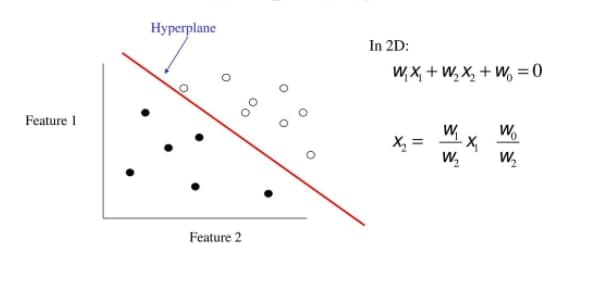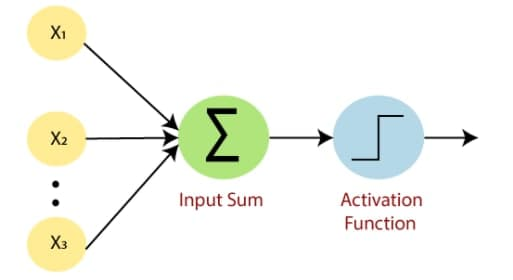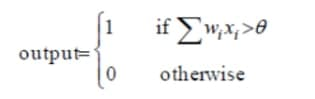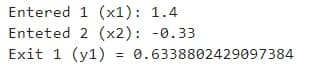It is the most fundamental of all neural network elements. It is the basic requirement to pursue before getting your hands on to more complex neural network problems further as this sets the tone. As many of you might know, the inspiration for the multi layer problem which is currently performing terrifically is derived from single layer perceptrons.

It is a machine Learning model which is a feed-forward network based on a threshold transfer function. SLP is the simplest type of artificial neural networks and can only classify linearly separable cases with a binary target (1 , 0).

This model of network was inspired from the working of neurons in human minds and how they react in order to perform certain given tasks. So this forms the structure of a network in order to build a network on linearly separable data.

This is how a linear separable data looks like after plotting.

A data is set to be linear separable when you can plot a hyperplane between two groups or classes which can separate them and to do this type of operation we use single layer perceptron.## Why SLP

SLP sounds unusual and complex but its working is too simple. We felt the need to come with this sort of neural network in order to perform linear separable problems using machines which will generate output with amazing performance and will provide more accurate solutions within no time especially when it comes to deal with huge amounts of data. By imitating these types of features in machines enhanced the wide range of machine learning applicability.

In order to boost the activity and to make outcome more close to perfect leads to formation of single layer perceptrons.

## How it works

In the first layer we can see that we are feeding the input to the network that acts as a perceptron to the networks. We usually set the first input as 1 value, also called a biased input.

One thing needs to be kept in mind is that we need to set the initial values of weights to 0 or a small random number. What it simply does is initialize the input to a direction that moves away from the origin in order to accelerate the feed forward network.The next thing is we assign some weights to those inputs with some activation function. These weights actually represent the strength between those perceptrons. Weights are assigned to input values also called nodes that have influence over each other so to generalize those impact factors we use weights. Once the inputs are being weighted they multiply with respective weight hence generating an equation. After the inputs are being weighted they are sent forward to the next step which is weighted sum. It actually sums up all the equations generated by all the input values and puts them all together to form neurons.

The end step is the output unit which is a weighted sum of inputs the result of being similar to the if, else function. To see if a single value is above the threshold(pre assigned values) , if yes then the value is 1, else 0. In the initial stage we told you that the target of SLP is to find out the similarities in data in order to separate them in binary classes, which is exactly what has been done here with 0 or 1.This is how the working of perceptrons takes place inside the neural network.

## Feed Forward Approach:

This method allows the network of inputs and outputs moves only in single direction and that is always forward hence the name Feed forward as the output of the current layer(input layer) is fed to the next layer as an input in order to perform the remaining operations.## Coding part

In the small section we have simply imported the libraries which are essential for the code which imports the java class and packages. In this case we are importing entire java and other library imports one addition static method for compiling

				
// here required libraries has been imported
import java.util.*
import kotlin.jvm.JvmStatic



Here we are defining a class which consist of constructors that are required and holds the data types for the values.

After that propogation function is being used to  to get the values with return exit values

				
// here class is been initialise with constructors that holds the data types for the values
class Neurona internal constructor(val x1: Double, val x2: Double, val w1: Double, val w2: Double) {
//Function propogation

val y1: Double
get() {
val wx: Double
val y1: Double
wx = x1 * w1 + x2 * w2 //Function propogation
y1 = Math.tanh(wx) //exit
return y1
}
}



Values from users has been entered prior for the working

				
//Values has been entered
val x1 = 1.4
val x2 = -0.33



Weights are being assigned to associate inputs and initial value is being assigned randomly close to the origin.
Finally the generated result has been printed

				
//values of weights has been randomly assigned
val w1 = Random().nextDouble()
val w2 = Random().nextDouble()
val n = Neurona(x1, x2, w1, w2)
println("Entered 1 (x1): $x1") println("Enteted 2 (x2):$x2")
println("Exit 1 (y1) = " + n.y1)



## Output## Limitations of SLP

Some of the main drawbacks of single layer perceptrons are that they can execute only a limited set of functions in the network, and one more important thing is the decision boundary of the dataset must be hyperplane or it may not be able to function.

The drawback of not being able to function on non linear data which covers all the real life world complex problems hence the invention of Multi Layer Perceptrons (MLP) happened since SLP is a linear classifier.